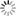# Episode List

OR

### Season 2

2 Oct. 1955
The Allentown IncidentBe the first one to add a plot.

9 Oct. 1955
Number Seven, Hangman's RowBe the first one to add a plot.

16 Oct. 1955
The Helping HandBe the first one to add a plot.

23 Oct. 1955
Pattern for ThievesBe the first one to add a plot.

30 Oct. 1955
Dark MemoryBe the first one to add a plot.

6 Nov. 1955
State of SiegeBe the first one to add a plot.

13 Nov. 1955
Stage FrightBe the first one to add a plot.

20 Nov. 1955
Relative StrangerBe the first one to add a plot.

27 Nov. 1955
Time BombBe the first one to add a plot.

4 Dec. 1955
EscapeBe the first one to add a plot.

11 Dec. 1955
A Sword Has Two EaglesBe the first one to add a plot.

18 Dec. 1955
The Big MistakeBe the first one to add a plot.

25 Dec. 1955
A Touch of ChristmasBe the first one to add a plot.

1 Jan. 1956
MutinyBe the first one to add a plot.

8 Jan. 1956
The Top of the MountainBe the first one to add a plot.

15 Jan. 1956
Suburban TerrorBe the first one to add a plot.

22 Jan. 1956
BetrayalBe the first one to add a plot.

29 Jan. 1956
The Battle of Hewitt HillBe the first one to add a plot.

5 Feb. 1956
All Through the NightBe the first one to add a plot.

12 Feb. 1956Be the first one to add a plot.

19 Feb. 1956
Till the End of TimeBe the first one to add a plot.

26 Feb. 1956
Paris VentureBe the first one to add a plot.

4 Mar. 1956
High StakesA former card shark tries to go straight, and his old police nemesis has him trap another card shark in a rigged game.

11 Mar. 1956
CareerA British woman, married to a German in Nazi-era Germany teaches English to school kids but is really an undercover secret agent for MI5, operating unknown to her pro-Hitler husband. At the brink of war, she must escape him and the regime to get information back to England.

18 Mar. 1956
A Thief There WasBe the first one to add a plot.

25 Mar. 1956
The House on Gellen StreetBe the first one to add a plot.

1 Apr. 1956
Two Falls for Satan